# Pool

The prism-shaped pool is 2 m deep with a bottom of the isosceles trapezoid with base dimensions of 10 m and 18 m and arm legs 7 m long and 5.7 m long. During the spring cleaning, the bottom and walls of the pool must be painted. How many m2 of paint should be used?

Correct result:

S =  163.8 m2

#### Solution: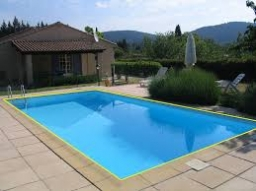We would be pleased if you find an error in the word problem, spelling mistakes, or inaccuracies and send it to us. Thank you!#### You need to know the following knowledge to solve this word math problem:

We encourage you to watch this tutorial video on this math problem:

## Next similar math problems:

• Apples 2James has 13 apples. He has 30 percent more apples than Sam. How many apples has Sam?
• Playground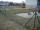Fencing square playground cost € 464; 1 meter cost € 19. What is the area of the playground?
• MO 2016 Numerical axisCat's school use a special numerical axis. The distance between the numbers 1 and 2 is 1 cm, the distance between the numbers 2 and 3 is 3 cm, between the numbers 3 and 4 is 5 cm and so on, the distance between the next pair of natural numbers is always i
• Rhombus 4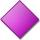Circumference of the rhombus is 44 cm, its height is 89 mm long. Calculate its content area.
• Isosceles trapezoid v3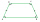In an isosceles trapezoid ABCD is the size of the angle β = 81° Determine size of angles α, γ and δ.
• CircleWhat is the radius of the circle whose perimeter is 6 cm?
• MineWheel in traction tower has a diameter 4 m. How many meters will perform an elevator cabin if wheel rotates in the same direction 89 times?
• Circle - simpleCalculate the area of a circle in dm2, if its circumference is 31.4 cm.
• Acreage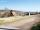Plot has a diamond shape, its side is 25.6 m long and the distance of the opposite sides is 22.2 meters. Calculate its acreage.
• A trapezoidA trapezoid 75 ft wide on top 85 ft on the bottom, the height is 120 ft. What is its area in the square yds?
• Trapezium 2Trapezium has an area of 24 square cms. How many different trapeziums can be formed ?
• FlowerbedIn the park there is a large circular flowerbed with a diameter of 12 m. Jakub circulated him ten times and the smaller Vojtoseven times. How many meters each went by and how many meters did Jakub run more than Vojta?
• GreenhouseGarden plastic greenhouse is shaped half cylinder with a diameter of 6 m and base length 20 m. At least how many m2 of plastic is need to its cover?
• Area of ditchHow great content area will have a section of trapezoidal ditch with a width of 1.6 meters above and below 0.57 meters? The depth of the ditch is 2.08 meters.
• Triangular prismCalculate the surface of a regular triangular prism with a bottom edge 8 of a length of 5 meters and an appropriate height of 60 meters and prism height is 1 whole 4 meters.
• The trench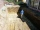Calculate how many cubic meters of soil needs to be removed from the excavation in the shape of an isosceles trapezoid, the top width is 3 meters, the lower width is 1.8 m, the depth of the excavation is 1 m, and the length is 20 m.
• Metal rails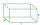Dad needs to improve the edges of the wooden boxes - to be reinforced with metal rails. How many cms of rails will he need if the box has the shape of a prism with the length of the edges 70cm 70cm and 120cm?